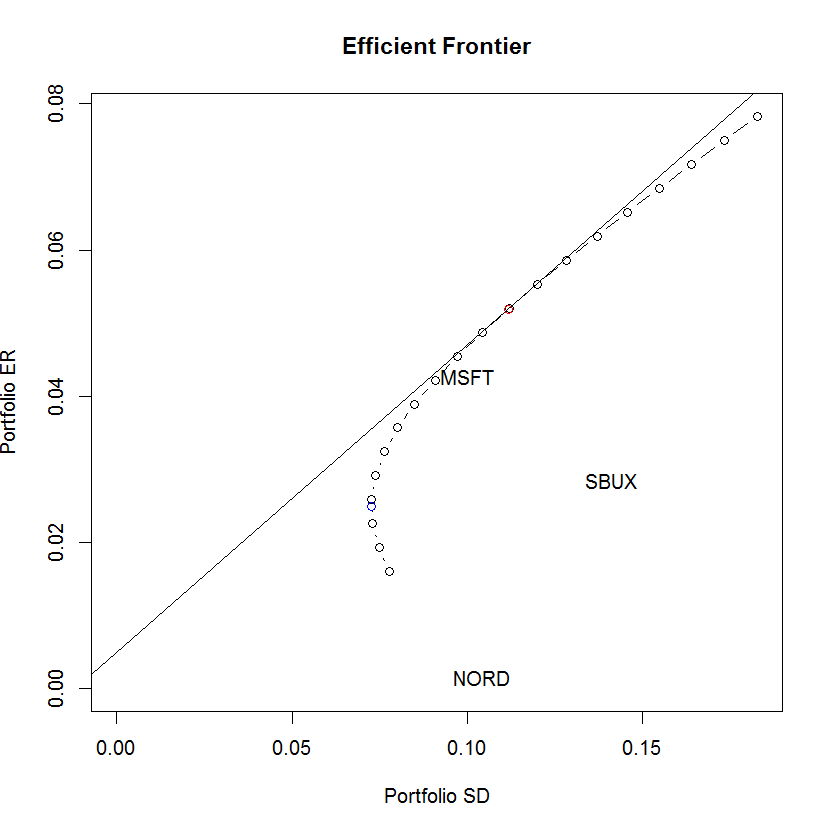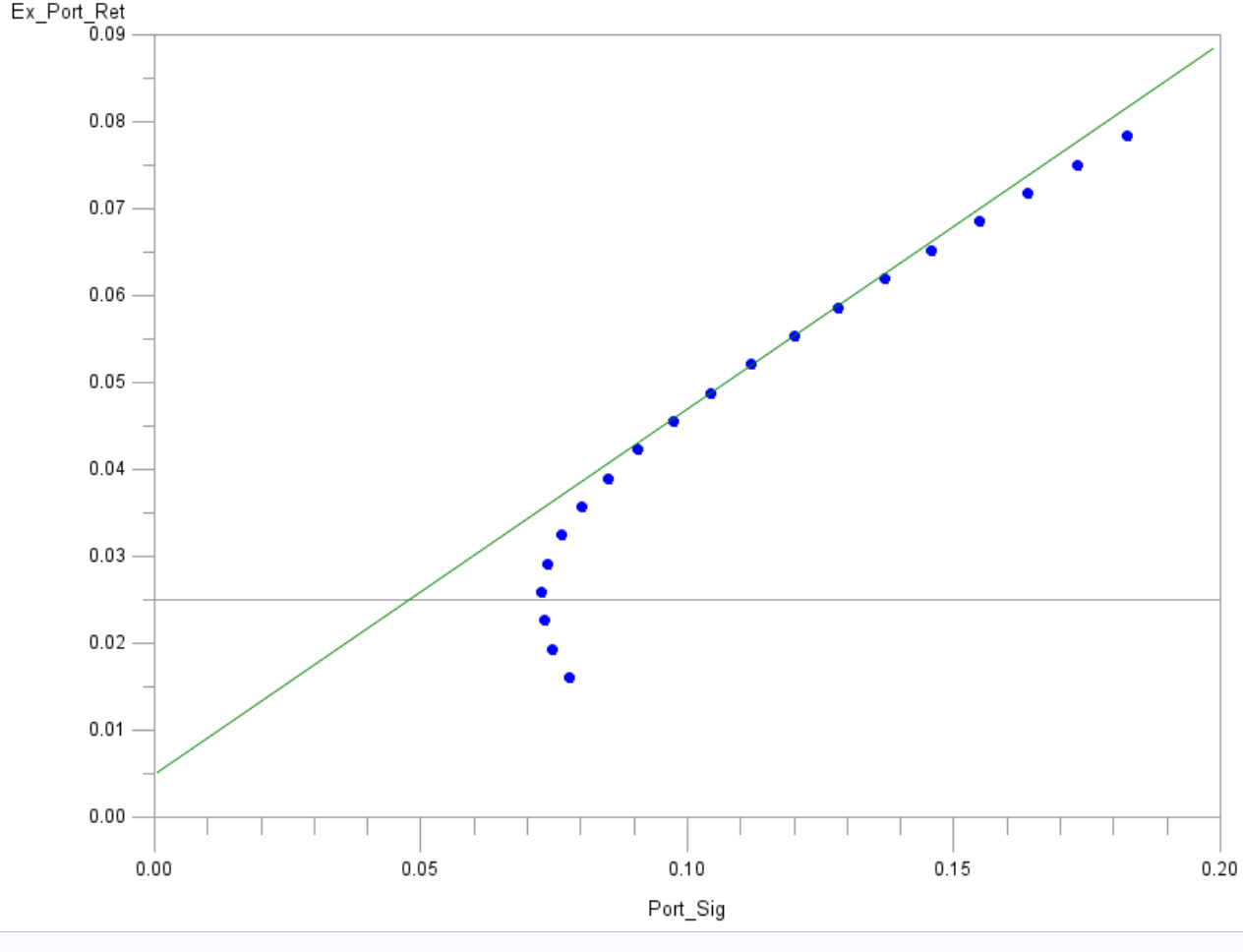## How to show name of a specific point on GPLOT

Hello to whomever can help

Please see the following R program and its graph output:

``````plot(ef, plot.assets=T)
points(gmin.port\$sd, gmin.port\$er, col="blue")
points(tan.port\$sd, tan.port\$er, col="red")
sr.tan = (tan.port\$er - rk.free)/tan.port\$sd
abline(a=rk.free, b=sr.tan)``````I can plot everything that on this R graph above on GPLOT except those names of points, MSFT, NORD and SBUX.

So what should I do?

The following is my GPLOT output and program. it does not have everything yet, such as title, but iknow how to put it up there.

Thank you!

``````goptions reset=all border;

symbol1 value=dot color=blue;
symbol2 value=none interpol=join color=green;
axis1 order=(0 to 0.09 by 0.01) offset=(0,0)
major=(height=2)
minor=(height=1);
axis2 order=(0 to 0.2 by 0.05) offset=(0,0)
major=(height=2)
minor=(height=1);

proc gplot data=proj.TanLine;
plot ex_port_ret*port_sig=1
Ex_tan_ret*Tan_port_sig=2/overlay
vaxis=axis1
vminor=1
haxis=axis2
hminor=4
vref=0.024891845;
run;
quit;``````## Re: How to show name of a specific point on GPLOT

Firstly, I would stongly advise to move to sgplot or graph template language, they are far more controllable.  As I don't use gplot anymore - moved to modern tech, I can only advise using sgplot/gtl.

What I would do is to create your graph, then have your point, defined in two seprate variables, and overlay that plot with marker labels turned on:

```data inter;
set your_data;
lab="MSFT";
labx=0.08;
laby=0.04;
run;
proc splot;
scatter x=y=mpg_city /  group=type markerchar=lab;
run;```

This does your graph, then overlays the scatter and places the text from LAB at the point LABX/LABY.  You can find plenty of examples of sgplot/gtl over at:

http://blogs.sas.com/content/graphicallyspeaking

Discussion stats